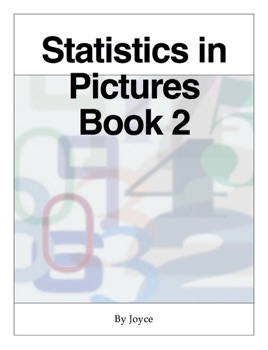## Description de l’éditeur

Statistics in Pictures Book 2 is the thirteenth book in the series, looking at working out the mean from frequency tables for discrete data, and from grouped frequency tables for discrete & continuous data. A working mean is introduced and applied to frequency tables for discrete data, and to a grouped frequency table using mid-interval values. Looking at the range, median, lower & upper quartiles and interquartile range for discrete data. At cumulative frequency tables, cumulative frequency curves and box plots for discrete & continuous data. Trends in line graphs, calculating moving averages, and drawing moving average trend line graphs. The correlation between two variables with scatter diagrams, and drawing lines of best fit to find relationships. Using Venn diagrams and listing possible outcomes for probabilities, including the probability scale and experimental probability. Listing outcomes of two events and plotting them in co-ordinate diagrams, in tables and possibility space diagrams. Looking at mutually exclusive and non-mutually exclusive events, listing possible outcomes, and using possibility space diagrams and Venn diagrams. Covering independent events by listing possible outcomes, and plotting points in diagrams. Finally we construct tree diagrams for two independent events, then for two dependent events, and finally for three independent events.

There are review questions at the end of sections.

The review questions require iOS 10 or later.